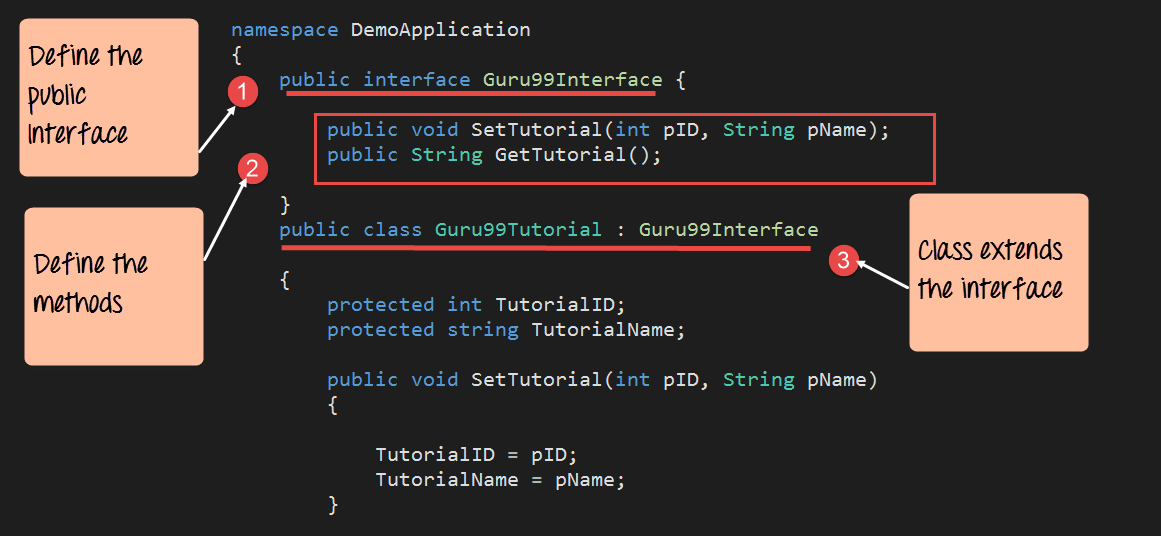# C# Interface

## What is an Interface Class?

Interfaces are used along with classes to define what is known as a contract. A contract is an agreement on what the class will provide to an application.

An interface declares the properties and methods. It is up to the class to define exactly what the method will do.

Let’s look at an example of an interface by changing the classes in our Console application. Note that we will not be running the code because there is nothing that can be run using an interface.

Let’s create an interface class. The class will be called “Guru99Interface.” Our main class will then extend the defined interface. All the code needs to be written in the Program.cs file.

```using System;
using System.Collections.Generic;
using System.Linq;
using System.Text;
namespace DemoApplication
{
interface Guru99Interface
{
void SetTutorial(int pID, string pName);
String GetTutorial();
}

class Guru99Tutorial : Guru99Interface
{
protected int TutorialID;
protected string TutorialName;

public void SetTutorial(int pID, string pName)
{
TutorialID = pID;
TutorialName = pName;
}

public String GetTutorial()
{
return TutorialName;
}

static void Main(string[] args)
{
Guru99Tutorial pTutor = new Guru99Tutorial();

pTutor.SetTutorial(1,".Net by Guru99");

Console.WriteLine(pTutor.GetTutorial());

}
}
}```

Code Explanation:-

Here, we explain the important sections of the code1. We first define an interface called “Guru99Interface.” Note that the keyword “interface” is used to define an interface.
2. Next, we are defining the methods that will be used by our interface. In this case, we are defining the same methods which are used in all of earlier examples. Note that an interface just declares the methods. It does not define the code in them.
3. We then make our Guru99Tutorial class extend the interface. Here is where we write the code that defines the various methods declared in the interface. This sort of coding achieves the following
• It ensures that the class, Guru99Tutorial, only adds the code which is necessary for the methods of “SetTutorial” and “GetTutorial” and nothing else.
• It also ensures that the interface behaves like a contract. The class has to abide by the contract. So if the contract says that it should have two methods called “SetTutorial” and “GetTutorial,” then that is how it should be.

Summary

• Summary
• An interface defines a contract which the class will comply with. The interface defines what are the operations that the class can perform.